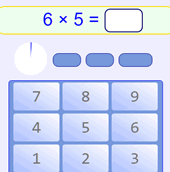# Multiplication Tips and Tricks

## Some Tips and Tricks

Everyone thinks differently, so just ignore any tricks that don't make sense to you.First of all: Memory is your best friend!

With the multiplication table in your memory you simply know that 3×5=15, 6×8=48 etc.

Memory is fast, too.

Math Trainer - Multiplication is designed to

## The Best TrickEvery multiplication has a twin, which may be easier to remember.

For example if you forget 8×2, you might remember 2×8=16. This way, you only have to remember half the table.

## Tricks by Number

2

add the number to itself (in other words, double it)
Example 2×9 = 9+9 = 18

4

double, then double again
Example 4×9: double 9 is 18, double 18 is 36

5

Cut in half, then times 10
Example: 5x6: Cut 6 in half to get 3, then times 10 for 30

Or times 10 then cut in half
Example: 5x9: 9 times 10 is 90, then cut in half for 45

Also the last digit goes 5, 0, 5, 0, ... like this: 5, 10, 15, 20, ...

6

when you multiply 6 by an even number, they both end in the same digit.
Examples: 6×2=12, 6×4=24, 6×6=36, etc

7×8

Think "5,6,7,8": 56=7×8

8

Double, double, double!
Example: 8×6: double 6 is 12, double 12 is 24, double 24 is 48

9

is 10× the number minus the number.
Example: 9×6 = 10×66 = 60−6 = 54

the ones digit goes 9, 8, 7, 6, ...: 9, 18, 27, 36, 45, ...
the tens digit goes  0, 1, 2, 3, ...: 9, 18, 27, 36, 45, ...

subtract one to get the tens digit, and the tens and ones digit together make 9
Example: 9×5: tens digit is 4, 4 and 5 make 9, so 45
Example: 9×8: tens digit is 7, 7 and 2 make 9, so 72your hands can help! Example: to multiply 9 by 8, hold your 8th finger down, and count "7" and "2", the answer is 72

10

put a zero after it
Example: 10×2 = 20

11

up to 11x9: just repeat the digit. Example: 11x4 = 44

for 11×10 to 11×18: write the sum of the digits between the digits
Example: 11×15 = 1(1+5)5 = 165

Note: this works for any two-digit number, but when the sum of the digits is more than 9, we need to"carry the one". Example: 11×75 = 7(7+5)5 = 7(12)5 = 825.

12

is 10× plus 2×
Example: 12×4 = 40+8 = 48

15

multiply by 10, then add half again
Example: 15×4 = 40+20 = 60
Example: 15×9 = 90+45 = 135

20

multiply by 10, then double
Example: 20×4 = 40+40 = 80
Example: 20×7 = 70+70 = 140

## Remembering Squares Can Help

This may not work for you, but it worked for me. I like remembering the squares (where you multiply a number by itself):

1×1 =1
2×2 =4
3×3 =9
4×4 =16
5×5 =25
6×6 =36
7×7 =49
8×8 =64
9×9 =81
10×10 =100
11×11 =121
12×12 =144

And this gives us one more trick. When the numbers we are multiplying are separated by 2 (example 7 and 5), then multiply the number in the middle by itself and subtract one. See this:

 5×5 = 25 is just one bigger than 6×4 = 24 6×6 = 36 is just one bigger than 7×5 = 35 7×7 = 49 is just one bigger than 8×6 = 48 8×8 = 64 is just one bigger than 9×7 = 63 etc ...